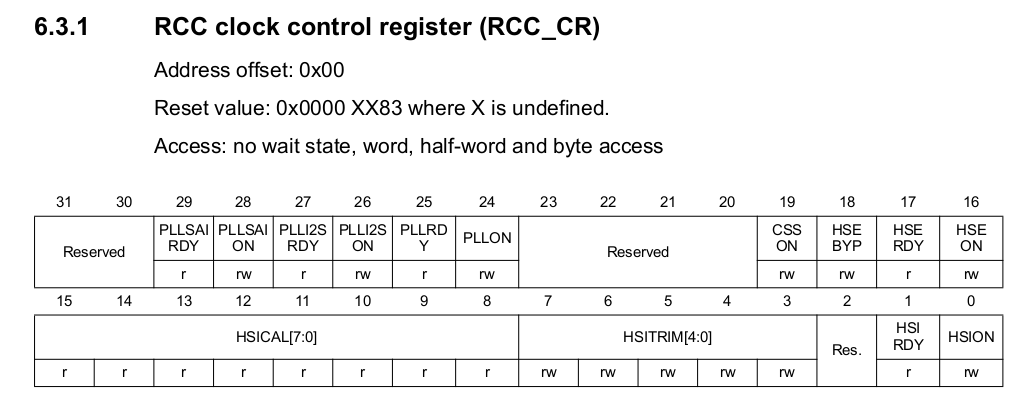# An introduction to ‘Bare Metal’-programming the Arm-Cortex-M4(R) MCUs – Lesson 3: Setting system clock

by Peter Baier (DK7IH)

With AVRs clocking was easy. Just set some fuse bits (but the right ones with the right values!!!!) , install external clock (optional) and go for it! With STM32s it is, as you might think, its alittle bit more complex.

### Various sources for the clock signal

In the STM32F4 MCU there are 3 possible way of generating a clock signal:

• Internal High-Speed-Oscillator (HSI) with 16MHz fixed frequency
• External High-Speed-Oscillator (HSE) normally from 8 to 25 MHz frequency (crystal based)
• PLL frequency derived from either HSI or HSE clock signal.

When you startup the system by supplying VDD the internal oscillator (HSI) is switched as clock source. Which clock you are about to use afterwards is defined in RCC->CR (Clock Control Register):You can see two bits that work identically for HSI respectively HSE 16 bits apart in the same register: An “ON”-bit and a corresponding “RDY”-bit. First you power the oscillator of your choice by setting the “ON” bit to “1”:

HSI on:

`RCC->CR |= (1 << 0);`

HSE on:

`RCC->CR |= (1 << 16);`

After being switched “on” these oscillators need a short period of time until they are ready for use. This is checked in a loop by polling the respective flag bit:

```while ((RCC->CR & (1 << 1) == 0); //Check HSI ready
while ((RCC->CR & (1 << 17) == 0); //Check HSE ready
```

### Using PLL as clock source

Besides using HSI oder HSE stand-alone, STM32F4 MCU allows the developer to apply an onboard PLL synthesizer to work as the primary clock source for the system. This might lead to a mac. clock frequency of 168 MHz. All settings are done by software, so you are not in danger, like with the AVRs, to “defuse” a controller. Software definition implies several steps because PLL setting involves definition of various parameters.

These parameters are named by the Alphabet, starting with PLLM, PLLN, PLLP and PLLQ.

Their frequencies are derived successively from the respective predecessor, the chain starts with PLLM. Provided we use 8 MHz HSE as input source, we have to modify PLLCFGR register. These steps are defined as:

```//Set PLLM
RCC->PLLCFGR &= ~0x3F; //1st reset bits
RCC->PLLCFGR |= 4;     //2nd define VCO input frequency
//= PLL input clock frequency (f.HSE) / PLLM with 2 ≤ PLLM ≤ 63
//-> f.VCO.in = 8MHz / 4 = 2MHz```

VCO input frequency is derived from HSE and divided by 4. This leads to f.VCO.in = 2 MHz which is fine because the boundaries are 1MHz <= f.VCO <= 2MHz (Ref. manual pages 163, 164).

Next is PLLN which is a multiplication and leading to VCO output frequency::

```//Set PLLN: PPLLN defines VCO out frequency
RCC->PLLCFGR &= ~0x7FC0; //1st Reset bits 14:6
RCC->PLLCFGR |= (100 << 6); //2nd define f.VCO.out =
//f.VCO.in * 100 = 200MHz```

With PLLP we define the PLL output frequency for modules like the USB interface. Certain limitations are to obeyed here, e. g. for USB frequency must be exactly 48MHz.

```//Set PLLQ. PLLQ = division factor for USB OTG FS, SDIO and random number generator clocks
RCC->PLLCFGR &= ~(0b1111 << 24); //Reset bits 27:24
RCC->PLLCFGR |= (8 << 24); //PLL-Q: f.VCO.out / 8 = 25MHz```

Nest step: We have to activate the PLL:

```RCC->CR |= (1 << 24); //Activate PLL, Bit 24
while ((RCC->CR & (1 << 25)) == 0); //Wait until PLL is ready Bit 25```

And finally the frequencies for the various bus systems and bridges must be set. For limitations see reference manual!

```                            //Division of clock signal for bus system
RCC->CFGR |= (0b1001 << 4) //AHB divider: 100MHz / 4 = 25 MHz
| (0b100 << 10) //APB1 divider: /2
| (0b100 << 13); //APB2 divider: /2```

Finally, not to be forgotten, PLL must be set as clock source for the MCU:

`RCC->CFGR |= 0b10; //Switching to PLL clock source`

Then you are ready to go!

Just another annotation: Onboard flash memory has to be configured as well. In Flash access control register (FLASH_ACR) the waitstates for FLASH memory have to be set. My rule of the thumb is:

• 1 WS for f=50MHz
• 2 WS for f>=100MHz
` FLASH->ACR |= 0b010; //2 wait state for 100 MHz`

If you want to experiment, write a short program that makes a LED blink and test it with various PLL settings up to 168 MHz. Just check if the program works. If the software hangs try to increase waitstates!

A full demo code for the Diymore STM32F4 (F407) board can be found on my Github repo: Link.

Hope you enjoyed the lesson. C U!

73 de Peter (DK7IH)